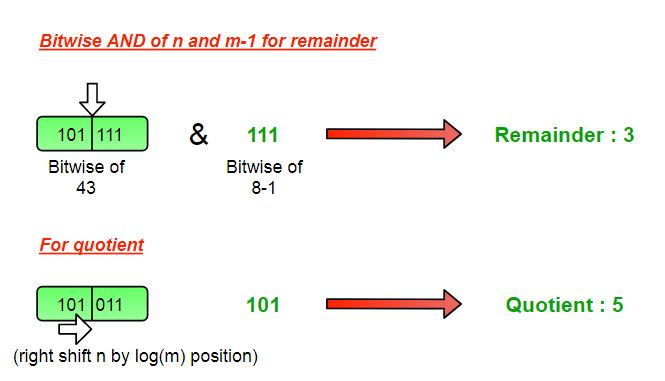# Quotient and remainder dividing by 2^k (a power of 2)

You are given an positive integer n as dividend and another number m (form of 2^k), you have to find quotient and remainder without performing actual division.

Examples:

```Input : n = 43, m = 8
Output : Quotient = 5, Remainder = 3

Input : n = 58, m = 16
Output : Quotient = 3, Remainder = 10
```

## Recommended: Please try your approach on {IDE} first, before moving on to the solution.

In this we are using bitwise representation of a number for understanding the role of division of any number by divisor of form 2^k. All numbers which are power of two includes only 1 set bits in their representation and we will use this property.
For finding remainder we will take logical AND of the dividend (n) and divisor minus 1 (m-1), this will give only the set bits of dividend right to the set bit of divisor which is our actual remainder in that case.
Further, the left part of the dividend (from the position of set bit in divisor) would be considered for quotient. So, from dividend (n) removing all bits right from the position of set bit of divisor will result into quotient, and right shifting the dividend log2(m) times will do this job for finding the quotient.

• Remainder = n & (m-1)
• Quotient = (n >> log2(m) )

Note : Log2(m) will give the number of bits present in the binary representation of m.## C++

 `// CPP to find remainder and quotient ` `#include ` `using` `namespace` `std; ` ` `  `// function to print remainder and quotient ` `void` `divide(``int` `n,``int` `m) ` `{ ` `    ``// print Remainder by  ` `    ``// n AND (m-1) ` `    ``cout <<``"Remainder = "` `<< ((n) &(m-1)); ` `     `  `    ``// print quotient by ` `    ``// right shifting n by (log2(m)) times ` `    ``cout <<``"\nQuotient = "` `<<(n >> (``int``)(log2(m))); ` `  `  `} ` ` `  `// driver program ` `int` `main() ` `{ ` `    ``int` `n = 43, m = 8; ` `    ``divide(n, m); ` `    ``return` `0; ` `} `

## Java

 `// Java to find remainder and quotient ` `import` `java.io.*; ` ` `  `public` `class` `GFG { ` `     `  `    ``// function to print remainder and ` `    ``// quotient ` `    ``static` `void` `divide(``int` `n, ``int` `m) ` `    ``{ ` `         `  `        ``// print Remainder by  ` `        ``// n AND (m-1) ` `        ``System.out.println(``"Remainder = "` `                        ``+ ((n) &(m-``1``))); ` `         `  `        ``// print quotient by right shifting ` `        ``// n by (log2(m)) times ` `        ``System.out.println(``"Quotient = "` `            ``+ (n >> (``int``)(Math.log(m) / Math.log(``2``)))); ` `    ``} ` `     `  `    ``// driver program ` `    ``static` `public` `void` `main (String[] args) ` `    ``{ ` `        ``int` `n = ``43``, m = ``8``; ` `         `  `        ``divide(n, m); ` `    ``} ` `} ` ` `  `// This code is contributed by vt_m. `

## Python 3

 `# Python 3 to find remainder and  ` `# quotient ` `import` `math ` `# function to print remainder and  ` `# quotient ` `def` `divide(n, m): ` ` `  `    ``# print Remainder by  ` `    ``# n AND (m-1) ` `    ``print``(``"Remainder = "``,  ` `                  ``((n) &(m``-``1``))) ` `     `  `    ``# print quotient by ` `    ``# right shifting n by  ` `    ``# (log2(m)) times ` `    ``print``(``"Quotient = "` `,(n >>  ` `          ``(``int``)(math.log2(m)))) ` ` `  `# driver program ` `n ``=` `43` `m ``=` `8` `divide(n, m) ` ` `  `# This code is contributed by ` `# Smitha `

## C#

 `// C# to find remainder and quotient ` `using` `System; ` ` `  `public` `class` `GFG ` `{ ` `    ``// function to print remainder and quotient ` `    ``static` `void` `divide(``int` `n,``int` `m) ` `    ``{ ` `        ``// print Remainder by  ` `        ``// n AND (m-1) ` `        ``Console.WriteLine(``"Remainder = "` `+((n) & (m - 1))); ` `         `  `        ``// print quotient by ` `        ``// right shifting n by (log2(m)) times ` `        ``Console.WriteLine(``"Quotient = "`  `                           ``+ (n >> (``int``)(Math.Log(m)))); ` `     `  `    ``} ` `     `  `    ``// Driver program ` `    ``static` `public` `void` `Main () ` `    ``{ ` `        ``int` `n = 43, m = 8; ` `        ``divide(n, m); ` `         `  `    ``} ` `} ` ` `  `// This code is contributed by vt_m. `

## PHP

 `> (int)(log(``\$m``, 2))); ` ` `  `} ` ` `  `    ``// Driver Code ` `    ``\$n` `= 43;  ` `    ``\$m` `= 8; ` `    ``divide(``\$n``, ``\$m``); ` ` `  `//This code is contributed by mits  ` `?> `

Output:

```Remainder = 3
Quotient = 5
```

Attention reader! Don’t stop learning now. Get hold of all the important DSA concepts with the DSA Self Paced Course at a student-friendly price and become industry ready.

My Personal Notes arrow_drop_upCheck out this Author's contributed articles.

If you like GeeksforGeeks and would like to contribute, you can also write an article using contribute.geeksforgeeks.org or mail your article to contribute@geeksforgeeks.org. See your article appearing on the GeeksforGeeks main page and help other Geeks.

Please Improve this article if you find anything incorrect by clicking on the "Improve Article" button below.

Article Tags :
Practice Tags :

Be the First to upvote.

Please write to us at contribute@geeksforgeeks.org to report any issue with the above content.